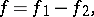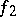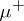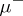# Jordan decomposition (of an endomorphism)

The Jordan decomposition of a functionof bounded variation is the representation ofin the formwhereandare monotone increasing functions. A Jordan decomposition is also the representation of a signed measure or a chargeon measurable setsas a difference of measures,where at least one of the measures (cf. Measure)andis finite. Established by C. Jordan.

## Contents

How to Cite This Entry:
Jordan decomposition (of an endomorphism). Encyclopedia of Mathematics. URL: http://encyclopediaofmath.org/index.php?title=Jordan_decomposition_(of_an_endomorphism)&oldid=21983
This article was adapted from an original article by M.I. Voitsekhovskii, V.L. Popov (originator), which appeared in Encyclopedia of Mathematics - ISBN 1402006098. See original article